/Introduction to quantum computing algorithms for the RAN (via Qpute.com)## Introduction to quantum computing algorithms for the RAN (via Qpute.com)

Management of the radio access network (RAN) is becoming increasingly efficient – performed by intuitive technologies, making optimized decisions in real time under unpredictable circumstances. However, as the volume and complexity of data processing increases, so too will the demand for increased computing power on the physical layer. To converge to a result within a limited time frame (i.e. execute machine learning algorithms or data processing at the physical layer), RAN management will soon require the computing power of a quantum processor.

In a previous blog post we introduced quantum computer technology and the future need of this type of specialized hardware to execute RAN functions. We foresee several use cases for quantum computing (QC) in the radio access network (RAN) including:

• Physical layer processing of the user data plane in the RAN (quantum Fourier transform and quantum linear solver)
• Clustering for automatic anomaly detection in network design optimization (quantum K-means algorithm)
• Prediction of the quality of user experience for video streaming based on device and network level metrics (quantum support vector machine)
• Database search at the data management layer (Grover’s algorithm)

Comprising quantum gates, specific quantum computing algorithms will be required to perform the RAN user data plane and management plane functionality. As of today, not all the classical algorithms are described in the quantum world and only a few of them have a quantum counterpart. We explore a selection of quantum computing algorithms for the RAN below.

1. User data plane processing in the RAN

• Quantum Fourier transform algorithm
The Quantum Fourier transform (QFT) is the quantum analogue of the discrete Fourier transform (DFT). DFT converts a discrete time sequence of a function to a discrete sequence of samples in the frequency domain. DFT can be implemented in computers by numerical algorithms. The most notorious example is the transformation of a sampled function in the time domain (ex. Sinusoidal) to a finite function in the frequency domain (ex. Dirac delta function). The input and output of the DFT equation are vectors of length N, the input and output of the QFT are quantum bit states of length n. Of particular interest here is that the relationship between length N and length n is . This means that an 8-bit vector (N=8) in the classical algorithm can be mapped to a 3-qubit state (n=3) in the quantum algorithm. This reduces the computational power by approximately 35 percent.
By using a series of Hadamard (H) gates and rotation gates we can compute the 3-qubit QFT (as shown in Figure 1). The |x1>, |x2> and |x3> states represent the 3-qubits. The Hadamard gates will transform the initial state of the qubit to a superposition state and by measuring any one of the qubits we will have a 50-50 chance of measuring 0 or 1. But once we have measured one qubit we will know exactly the state of the other one.  The Rotational (R) gates usually act on one qubit, however, in this case, the rotational gates act on two qubits. One qubit will take the control, the other one will have a phase-shift of an angle ? according to the control. This is done by leaving the |0> state unchanged and rotating the |1> state by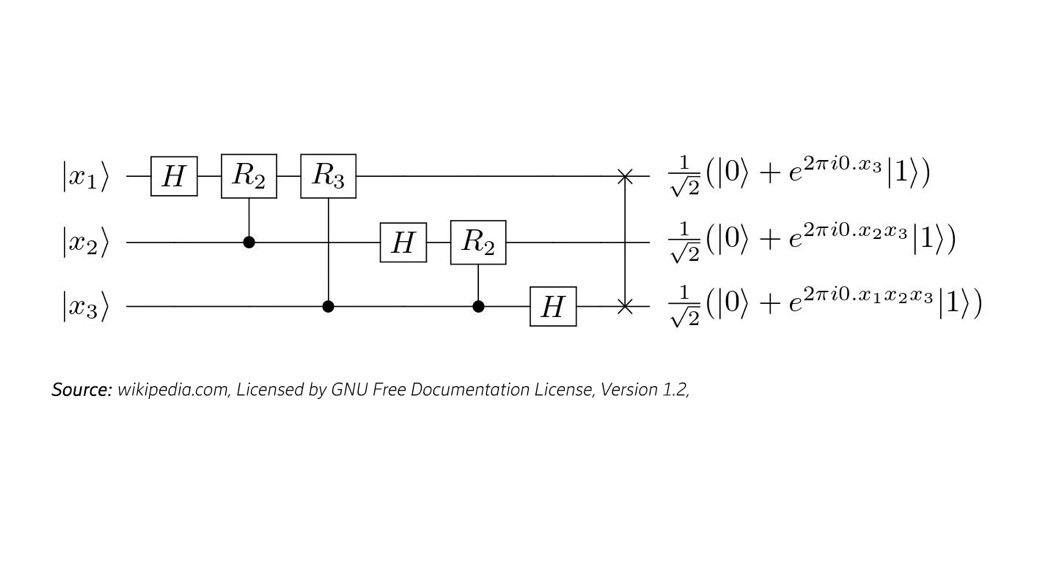Figure 1. Quantum circuit for 3-qubit QFT

• Quantum linear equation solver algorithm
Prediction of the quality of user experience for video streaming based on device and network level metrics will use algorithms based on linear equation solvers. The HHL algorithm, named after its authors, Aram Harrow, Avinatan Hassidim, and Seth Lloyd, is the quantum algorithm that solves a system of linear equations. For a given system of N linear equations with N unknowns, HHL can find satisfying , where A is an NxN matrix, and  is a unit vector with size Nx1.
The HHL algorithm can provide exponential speedup for the classical task. HHL is also considered a fundamental quantum algorithm, as it is the base to realize many other quantum machine learning algorithms, including quantum support vector machine (for classification problems) and quantum principle component analysis (for dimension reduction).

The quantum circuit for the HHL algorithm and implementation can be seen in Figure 2 below.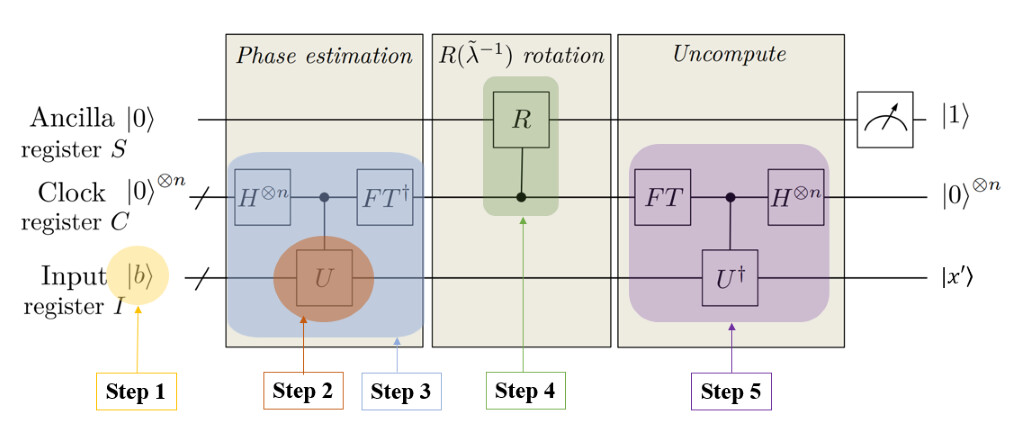Figure 2. Quantum circuit for HHL algorithm. FT: Fourier Transform; U: rotation gate; H: Hadamard gate; R: rotation gate.

2. Quantum clustering algorithm for the RAN management plane

K means is an unsupervised clustering algorithm that groups together n observations into k clusters making sure intra-cluster variance is minimised. The quantum version of the K means algorithm hopes to achieve exponential speedup over its classical counterpart and, at the same time, provide results of comparable accuracy. A study performed by Schuld et al. proposes a Quantum Interference Circuit that can perform distance-based classification. The full circuit as proposed by the paper is shown in figure 3. Again the algorithm’s mathematical operations can be expressed in terms of quantum gates (Hadamard, Rotation, Pauli). The X gate here is a single qubit gate, known as Pauli X-gate or quantum NOT gate. It produces a rotation around the x-axis by π radiants and maps |0> to |1> and |1> to |0>. In figure 3, we also have a controlled-NOT gate, represented by a NOT gate (circle with the plus sign inside) and a dot joined with a vertical line. This gate acts on 2 or more qubits and will perform the NOT operation on a second qubit, if and only if, the first qubit is in the |1> state. Finally, the double CNOT gate (Toffoli gate or CCNOT), represented by a circle with a plus sign and one line joining two dots, is a 3-qubit gate that will apply a NOT gate on the third qubit provided that the other two qubits are in the |1> state. These series of gates and operations are used for the quantum version of the K-means algorithm, thus to perform quantum clustering.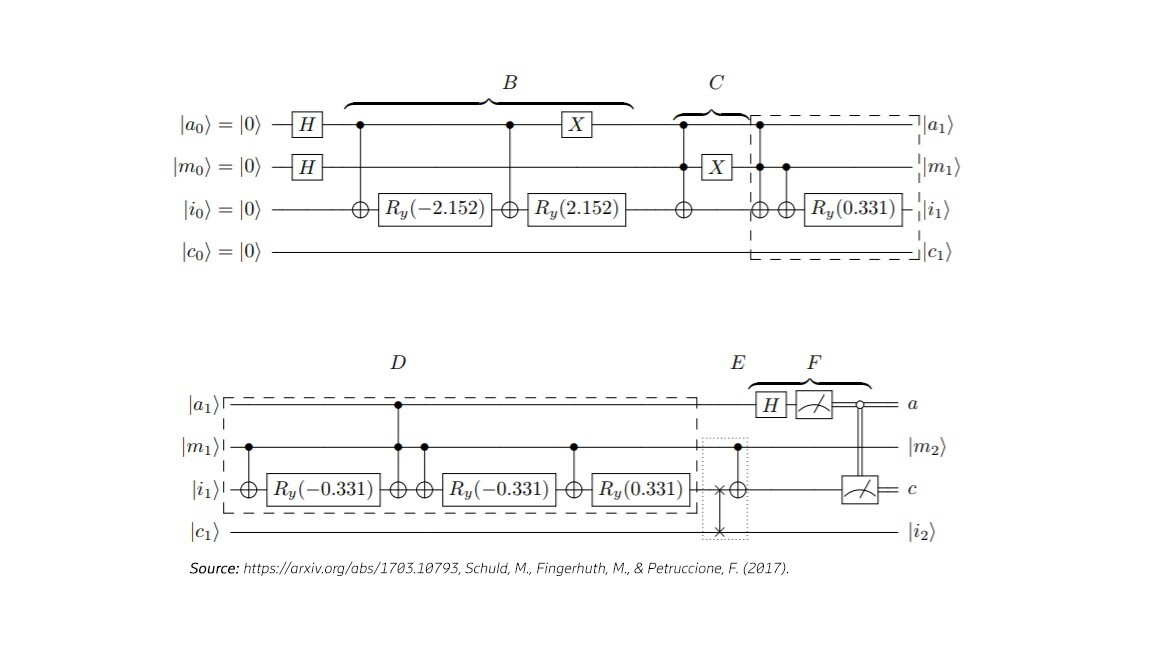Fig 3. Quantum Interference Circuit to perform distance-based classification (3)

3. QSVM algorithm for the RAN management plane

Support vector machine (SVM) is a supervised machine learning technique for solving classification or regression problems. It can classify vectors into two-subgroups. QSVM is a quantum algorithm redesigned for implementing the original classical algorithm on quantum computers, and it can quadratically or exponentially speed up the original classical algorithm. There are two methods for implementing QSVM. One is based on Grover’s Search algorithm, with quadratic speedup; the other is based on HHL algorithm (see figure 4), with exponential speedup.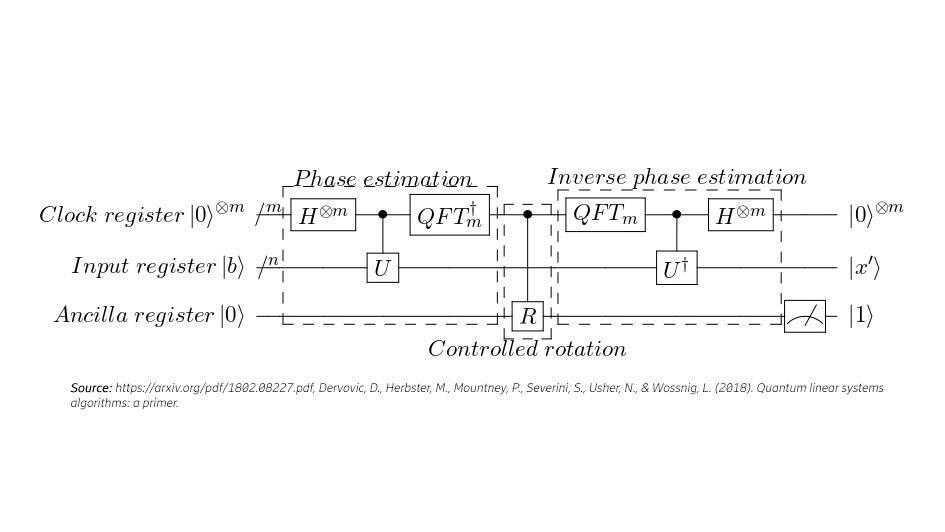Figure 4. Quantum circuit for the SVM algorithm based on the HHL algorithm.

4. Grover’s algorithm for the RAN management plane

Another algorithm we are interested in is a database search. Lov K. Grover presented in 1996 what he considered the fastest possible quantum mechanical algorithm. Grover’s algorithm finds out the n value with N number of unsorted elements in a database. To search for this n number, any classical algorithm (probabilistic or deterministic) takes N number of operations as it checks and performs a step by step process of computational complexity O(N). Using Grover’s search, the algorithm has a computational complexity O(√N) instead. Note the square root difference between the two algorithms, thus, Grover’s algorithm can search a n number in a database with reduced complexity and execution time. This is based on the principle that by adjusting the phase of various operations, successful computations reinforce each other while others interfere randomly.  Grover’s algorithm uses two main operations, the Hadamard transform and the conditional phase shift operation. Both are relatively easy to implement as compared to other quantum computing gates. Figure 5 shows the quantum circuit functional blocks of 3-qubit Grover’s algorithm.

In a first stage, an initialization process (see blue box in the figure below) will set all the qubits in superposition. This is done by applying the Hadamard gate to each qubit. After this operation the amplitude of each state is . In a second stage (see beige box in the figure below), there is an oracle function that performs a phase flip of a desired quantum state (for example |111>). The phase flip inverts the amplitude of this state making it -0.35. All other states are left unaltered. After the oracle, an amplification stage (see green box in the figure below) performs an inversion about the average of the amplitudes. As the target state’s amplitude is inverted, the flip causes the target state’s amplitude to increase and the other ones to decrease. The average amplitude is ( The |111> state differs from the average by 0.2625 – (-0.35) = 0.6125. An inversion around the average amplitude results in an amplitude for |000> of 0.2625+0.6125 = 0.875. Finally, the qubits are measured, and an output is given (See yellow box in the figure below).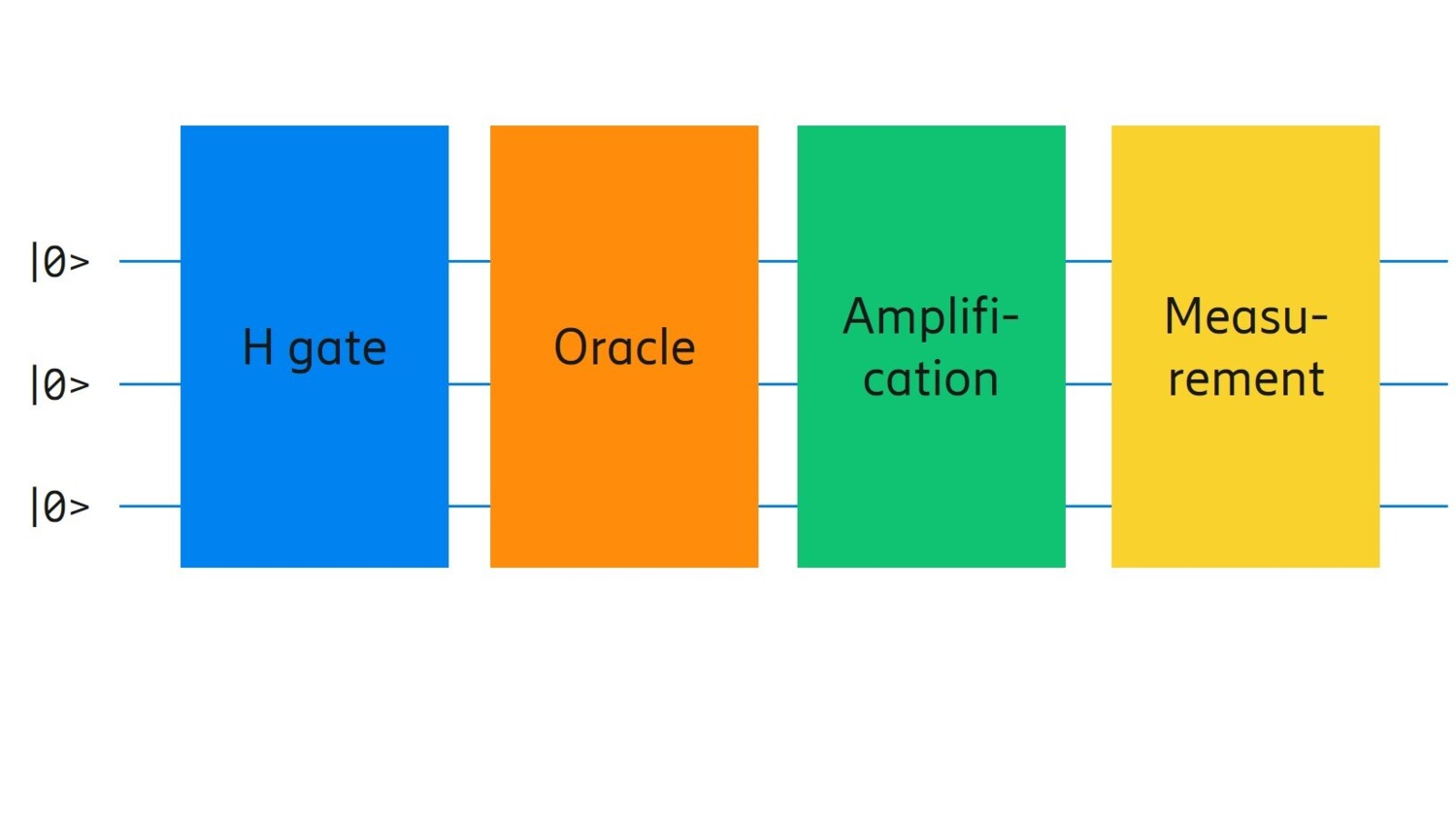Figure 5. Three qubit implementation of Grover’s algorithm after Grover’s published work.

When any of these algorithms are executed on available quantum hardware, we experience a limitation in the number of qubits, gates, and operations to perform. The main limitation in this regard is the coherence time of the qubits. Coherence time is the amount of time a qubit can maintain its superposition state without collapsing to a defined state. Current quantum computers have qubits with very low coherence times. Thus, quantum computation must finish before the computation collapses. In pursuit of having better results on  near term noisy quantum computers, shorter depth circuits are desired. In one specific example, we optimised the quantum support vector machine classification circuit by reducing the number of gates from 22*m (where m is the number of test data points) to 20. This, in turn, allows us to decrease the run time of the QSVM algorithm from 6.145s to 0.0324s; (189 times). In another example, we reduced the circuit depth of the quantum K means by loading interfering copies of the input vectors with the same relative angular difference. This allows us to classify 100 random data points into two clusters (see Figure  6).Figure 6. Left: 100 data points are classified into two clusters. Right: clustering normalized to the unit circle. Both results were obtained using IBM’s quantum chip IBMQX2.

Current and upcoming noisy quantum computers are subject to environmental error. Qubits have low coherence times and their superposition is quickly lost. The algorithms presented here are quantum algorithms examples with shallow depth quantum circuits and want to provide a guideline of how RAN algorithms need to be designed and how they perform when executed in noisy intermediate scale quantum computers. In a further blog post we will visit the timeline and the possible scenarios for deploying RAN quantum algorithms.